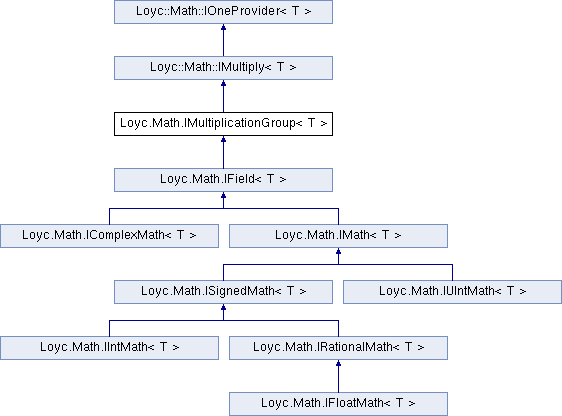Enhanced C# Language of your choice: library documentation
Loyc.Math.IMultiplicationGroup< T > Interface Template Reference

This defines a Group with the operation *, the neutral element One, the inverse Inverse and an operation / that is defined in terms of the inverse. More...

Source file:
Inheritance diagram for Loyc.Math.IMultiplicationGroup< T >:## Remarks

This defines a Group with the operation *, the neutral element One, the inverse Inverse and an operation / that is defined in terms of the inverse.

Axioms that have to be satisified by the operations: Commutativity of multiplication: Multiply(a,b)=Multiply(b,a) for all a,b in T Associativity of multiplication: Multiply(Multiply(a,b),c)=Multiply(a,Multiply(b,c)) Inverse of multiplication: Multiply(a,Inverse(a))==One for all a in T Divison: Divide(a,b)==Multiply(a,Inverse(b)) for all a in T Neutral element: Multiply(One,a)==a for all a in T

Shl and Shr (shift left/right) operations are commonly thought of as binary operations, but some algorithms need to multiply numbers by powers of two and want to do so efficiently, while still supporting floating-point types. Therefore it makes sense to offer Shl ("multiply by a power of two") and ShiftRight ("divide by a power of two") operators as part of the multiple/divide interface, not just IBinaryMath<T>. Even floating-point types can support these two operations efficiently by directly modifying the exponent part of the floating-point representation.

## Public Member Functions

Div (T a, T b)

Shl (T a, int amount)

Shr (T a, int amount)

MulDiv (T a, T mulBy, T divBy)Public Member Functions inherited from Loyc.Math.IMultiply< T >
Mul (T a, T b)Properties inherited from Loyc.Math.IOneProvider< T >
One` [get]`